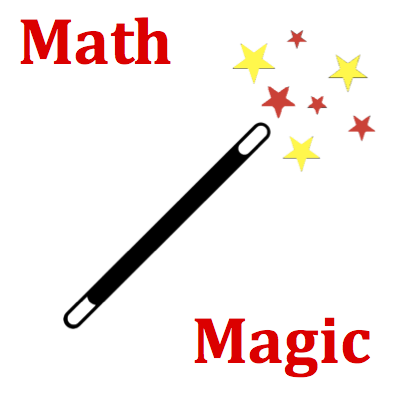# Forces in Two Dimensions - Mission F2D1 Detailed HelpA vector is directed at a direction of 110 degrees (as measured counterclockwise from east). Such a vector would have two components. The ____ component would be greater than the ____ component.   (Note: Numbers are randomized numbers and likely different from the numbers listed here.)Definition of Vector Component A vector component is a projection of a vector onto the horizontal or vertical axis.Counterclockwise from East Convention for Vector Direction The direction of a vector is often expressed using the counterclockwise (CCW) convention. According to this convention, the direction of a vector is the number of degrees of rotation which the vector makes counterclockwise from East.The components of a vector are often represented on a diagram by constructing a right triangle about the vector such that the vector is the hypotenuse of the right triangle. The components are then the legs of the right triangle. (You have likely seen such diagrams and might make an effort to sketch one now.) If the vector is a northwest vector, then it has components stretching west and north. The west and north components are simply the west and north legs of the triangle which has been created from the northwest vector.   Trigonometric functions can be used to determine the precise magnitude of the legs of these triangles. If the angle Thetais the counterclockwise angle of rotation between the eastern axis and the vector, then the x-component can be calculated using the cosine function. The y-component can be calculated using the sine function.   Ax= A • cosineΘ Ay= A • sineΘ   where Θ = counterclockwise angle rotation of vector A from east.